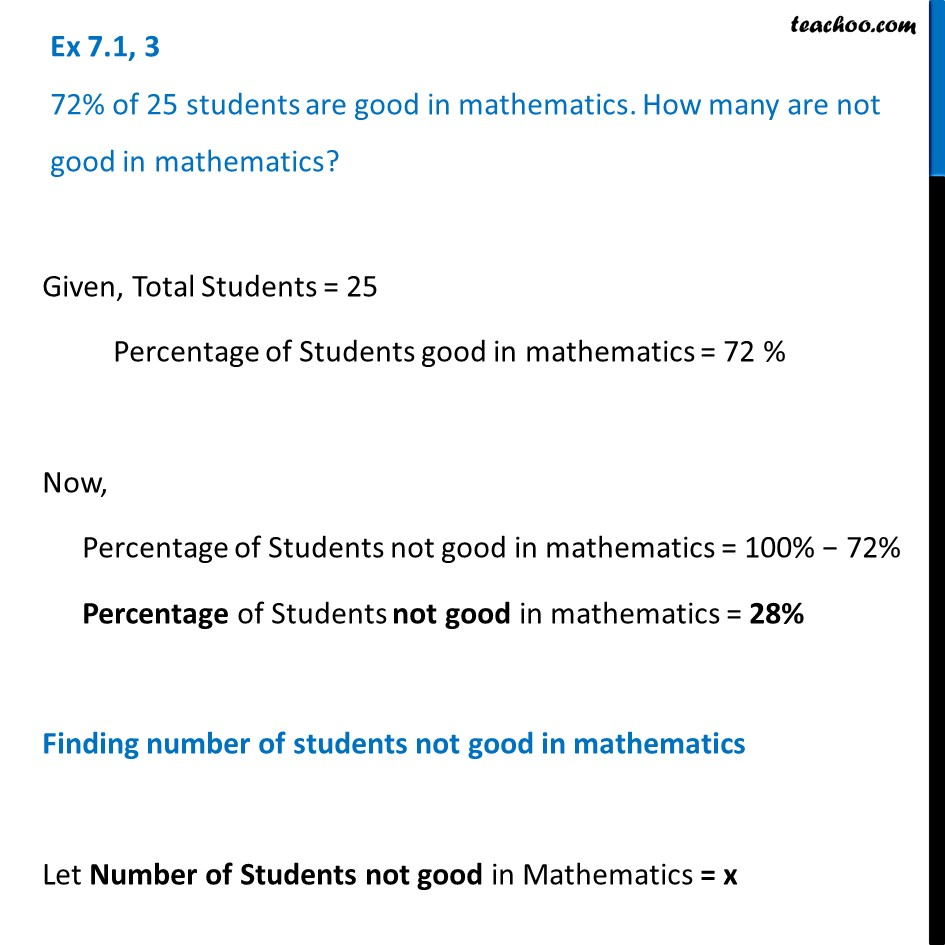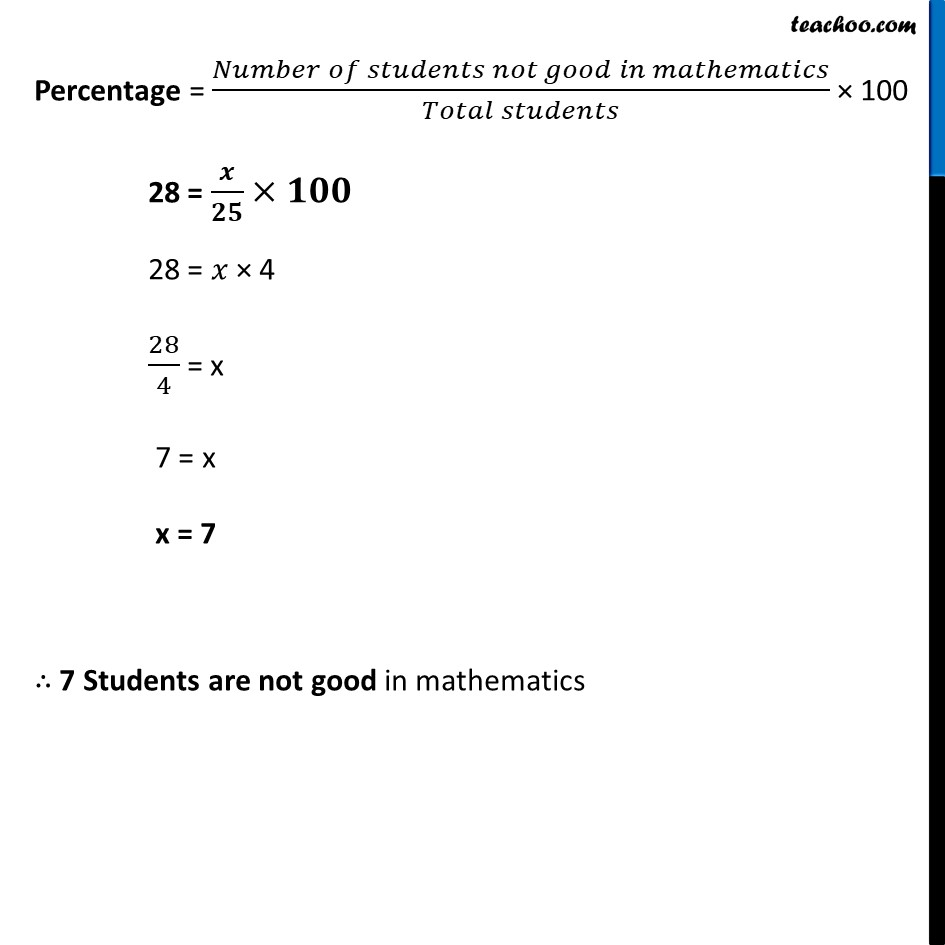Ex 7.1

Chapter 7 Class 8 Comparing Quantities
Serial order wiseLearn in your speed, with individual attention - Teachoo Maths 1-on-1 Class

### Transcript

Ex 7.1, 3 72% of 25 students are good in mathematics. How many are not good in mathematics?Given, Total Students = 25 Percentage of Students good in mathematics = 72 % Now, Percentage of Students not good in mathematics = 100% − 72% Percentage of Students not good in mathematics = 28% Finding number of students not good in mathematics Let Number of Students not good in Mathematics = x Percentage = (𝑁𝑢𝑚𝑏𝑒𝑟 𝑜𝑓 𝑠𝑡𝑢𝑑𝑒𝑛𝑡𝑠 𝑛𝑜𝑡 𝑔𝑜𝑜𝑑 𝑖𝑛 𝑚𝑎𝑡ℎ𝑒𝑚𝑎𝑡𝑖𝑐𝑠)/(𝑇𝑜𝑡𝑎𝑙 𝑠𝑡𝑢𝑑𝑒𝑛𝑡𝑠) × 100 28 = 𝒙/𝟐𝟓×𝟏𝟎𝟎 28 = 𝑥 × 4 28/4 = x 7 = x x = 7 ∴ 7 Students are not good in mathematics